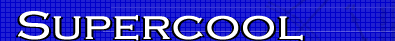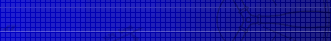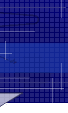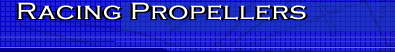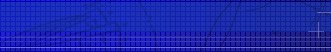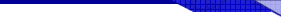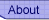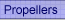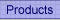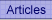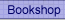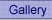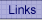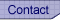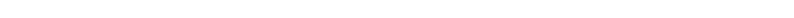﻿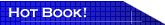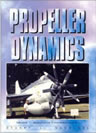Propeller Dynamics Essential reading for model aircraft contest fliers. This is the only book on the market explaining propeller theory in non-mathematical terms. A rattling good read, I know, I wrote it. Read more...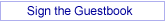## Analytical Design of Propellers - part 2 Determination of C1

By Joe Supercool

In my previous article, “Analytic design of propellers - part 1”, I referred to the need for a value of the lift coefficient Cl derived from the Joukowski relationship; ie, Cl derived from circulation theory. In my initial calculations I tried equations given by Larrabee and Liebeck and Adkins. These equations did not give realistic values when matched to my known propeller performance data, so I was forced to look further. The Larrabee paper was “Propeller Design and Analysis for Modellers”, NFFS, 1979 (ref.1).

I found that Larrabees’ derivation was riddled with “light loading” approximations, which tended to remove the physics. Because I already know the performance of my propellers, in particular the interference factors, I am not hampered by some of Larrabees’ constraints.

Returning to Larrabees’ “Analytic design of propellers having minimum induced loss”, NFFS 1977 (ref.2), I found Figure 2, p91, which is a sketch of the motion of a helical vortex filament in the developed slipstream.

Ye Gods and little fishes, a what? Well, if we think in terms of a single blade element, then the path of that element influences an annulus of width say r to r + dr, where r is the radial station and dr the width of the blade element. Inscribed on the surface of the annulus we can imagine the helical path of the air displaced by the blade element. This displaced air is part of the vortex sheet shed by the entire propeller blade. Let us call this the “helical vortex filament”.

The displaced air of the vortex filament has velocity normal to the vortex sheet at station r of value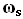and with components axially of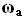and radially of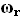.

Because we have only a finite number of blades, which do not intersect all the air coming into the prop, we need to invoke Prandtl’s vortex sheet spacing correction, via the quantity F. Then we may write, for a vortex filament at station r to r + dr: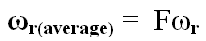From the first term in equation (8) (ref.1, p11), we can write: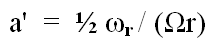so that: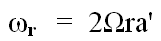and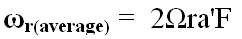Moving to the first term in equation (1) (ref.1, p10) yields: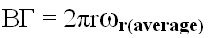and substituting gives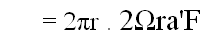whence the circulation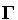is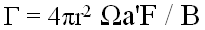From equation (16) (ref.2, p92),is also given by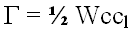Equating the two expressions foryields finally for the lift coefficient Cl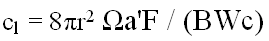From the geometry of Larrabee’s Figure 4 (ref.2, p93) and the known performance of our propeller we can get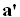and W by varying the angle of attack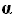until Cl lies on the lift slope curve at the design lift coefficient.

This completes the exercise, and hopefully yields a value of Cl which matches the empirical data.

In the above, B is the number of blades, C the chord of the blade element at radius r, W the inflow vector after allowing for downwash,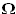the angular velocity andthe torque plane interference factor.